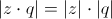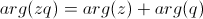# Multiplying Complex Numbers, Day 4 of 4

1 teachers like this lesson
Print Lesson

## Objective

SWBAT use the unit imaginary number and the field axioms to multiply complex numbers. SWBAT represent and interpret multiplication of complex numbers in the complex number plane.

#### Big Idea

Practice helps students uncover hidden patterns and discover simple rules.

## Reviewing Homework & Setting the Stage

10 minutes

Class begins by reviewing the answers to the first problem from Practice 3, which was last night's homework.

It's likely that some students will have forgotten (in a mere 24 hours) how to calculate the argument and distance.  Another common error is to simply record product of the complex numbers as the distance.  A good way to clear this us is to ask a student to come to the board to demonstrate how to solve one of the problems.  By the end, everyone should have three numbers plotted in the complex plane, three distances, and three arguments computed.

## Multiplication Practice

25 minutes

Now, the remainder of class is devoted to completing Practice 3 and Practice 4.  Students are free to work individually or in small groups, as long as they are on task.  They will be checking their answers against one another, and I will be circulating among them offering encouragement and answering questions.  The students should quickly notice that they're once again given the same numbers for z that they had on Practice 1, so they can copy over their solutions from that sheet.

I'll also encourage them to keep an eye out for those patterns we had noticed in the previous lesson. (MP 8)  Do they hold true?  If not, perhaps you've found a real counter-example, or perhaps you should double-check your calculations!  As students begin to tell me that these patterns really do seem to be always true, I begin to challenge them to find a way to express them precisely. (MP 6)  It's important to be able to describe the pattern in a way that will be clearly understood once we put it into writing.

One suggestion: encourage your students to plot the numbers in the plane before trying to calculate the argument.  They must know which quadrant their number lies in before they can figure out the correct angle.

As you begin to think about how much practice is enough, consider this video.

As an extension for advanced students, you may consider assigning the Stars problem.  Here the complex numbers form a nice star shape in the plane.  As students add and multiply, the star is translated, reflected, and dilated around the plane.

## Describing Patterns

10 minutes

The homework tonight is to complete the rest of Practice 3 and Practice 4.

Before class ends, however, it's important to once again summarize the day's progress.  Did those patterns we identified yesterday remain true?  Has anyone found a way to put them into words?  I'll ask for students to share the patterns they've identified, and then I'll rely on the rest of the class to help articulate those patterns precisely.  Here are my three goals for this conversation:

1. Multiplying a complex number by anything other than a positive real number causes it to rotate.  Notice that this includes multiplying by -1, which is i*i after all.
2. Because of similar triangles, multiplying by a real number causes the distance to increase by that factor.  Many students also explain this in terms of slope; the real and imaginary parts have both been scaled by the same factor but their ratio remains the same.
3. "Distances are multiplied, but arguments are added."  That is,and.

Bear in mind that it's not important that everyone understand why this pattern or rule is true.  For Algebra 2, it's enough that students can correctly multiply the complex numbers and can compute distances and arguments.  The rest is icing on the cake.